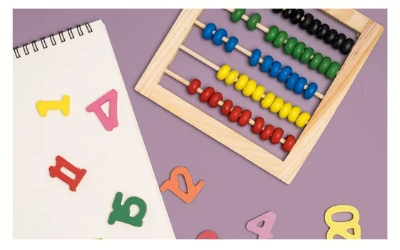# Math Riddles With Answers: Can You Solve These Two Basic Math Problems?

This math riddle has two of the easiest and most basic arithmetic problems. Test your basic math skills here by solving this math riddle.Math Riddles With Answers: Can You Solve These Two Basic Math Problems?

Welcome to another one of our math riddle series. You probably know by now why solving math riddles are great for you and your mind. So, here we are with another set of math riddles with answers waiting for you.

Before you think this is going to be tough and run away, stop! We have prepared two of the simplest and basic math riddles today.

So give these math riddles a try and test how good your basic mathematical skills are.

## Math Riddles With Answers: Can You Solve These Two Basic Math Problems?

Math Riddle #1

Here Are 5 Math Riddles On Reasoning To Prepare You For Your Exams

Math Riddle #2

All the best!

Bored Of Tough Problems? Here Are Fun Math Riddles For Adults.

Math Riddle #1

Ans.

Math Riddle #2

Ans. 86. When 86 is turned upside down and flipped, it becomes 98, which is 12 more than 86.

We hope you liked this.

Math Riddles For Exams: 5 Mind-Breaking Problems On Profit & Loss.

Get the latest General Knowledge and Current Affairs from all over India and world for all competitive exams.
खेलें हर किस्म के रोमांच से भरपूर गेम्स सिर्फ़ जागरण प्ले पर